Part 2: Calibration & Imaging of J1849+3024

This section expects that you have completed part 1 of the EVN Continuum tutorial. The result of that section is that you should have learnt the basics of fringe-fitting, inspection of data and bandpass calibration. In the following section, we shall look at refining our calibration and imaging basics. By the end of this section we should have an image of our target sources.

While in the previous section we used CASA interactively, this section will rely on scripting you own calibration script. Generating a script to calibrate your data is advantageous as you can reproduce the calibrated data in the future in case of accidental deletion or corruption of these data. We would advise that if you are unfamiliar with python then you should sit near someone who is, or, if you are working remotely, take a look at the computing courses on these webpages.

1. Data and supporting material

For this section, you will need the scripts contained in EVN_continuum_pt2_scripts_casa6.tar.gz (download link is on the workshop homepage) and the $uv$ data set - 1848+283_J1849+3024.ms - from part 1. Put these two files into different folders so that you can distinguish between parts 1, 2 and 3 of this tutorial and un-tar the scripts. You should have the following in your folder:

• 1848+283_J1849+3024.ms - partially calibrated $uv$ data. Note that if you find weird results please download the $uv$ data (EVN_continuum_pt2_uv_casa6.tar.gz) using the link on the homepage.
• NME_J1849.py.tar.gz - complete calibration script to use as a reference or if you get stuck.
• NME_J1849_skeleton.py - calibration script we will input to.

2. Scripting in CASA

The CASA calibration package allows you to create your own scripts which can be executed in the CASA prompt or from the command line. You will remember from part 1 that we executed some scripts (e.g. append_tsys.py & gc.py) in the command line using the -c argument. In this section, we shall be executing scripts from within the CASA prompt.

To begin do the following:

• Open both of the files NME_1849.py and NME_1849_skeleton.py using a text editor e.g. gedit. NME_1849_skeleton.py is the file which we will be inputting our commands into, while we shall use NME_1849.py as the guide. Please do not copy and paste from one to the other as you won't learn anything!
• Open a CASA interactive prompt by typing casa into the command line.

Take a look at the NME_1849_skeleton.py file. This file provides an example of a CASA calibration script. The script is written such that only a subset of commands are executed at once (note that there are many ways to do this!). This is advantageous as we can test and run small parts of the scripts to ensure that we are doing each part correctly rather than waste time executing all the commands all at once only to find many mistakes along the way. Let's take a look at the preamble to see how this is done.

Lines 33-37:

### Inputs ###########
target='J1849+3024'
phscal='1848+283'
antref='EF'                  # refant as origin of phase
######################

One of the main advantages with scripting is that we can set variables to be used across the entire script. While for many data sets, the calibration may remain similar, these variables are often different between data sets but are common within the script, e.g. targets, phase cals, experiment names and antennas. By setting such variables it means we only have to adjust a few lines of code to adapt these scripts to other data.

Lines 40-78:

thesteps = []
step_title = {1: 'Image phase cal',
2: 'Obtain image information',
3: 'FT phase cal to generate model',
4: 'Refine delay calibration',
5: 'Refine phase calibration',
6: 'Apply all calibration to phasecal',
7: 'Re-image phasecal',
8: 'Phasecal selfcal in amp and phase',
9: 'Apply solutions to phasecal',
10: 'Re-image phasecal with selfcal done',
11: 'Apply solutions to target',
12: 'Split target from ms',
13: 'Image target w/ calibration from phase referencing',
14: 'Look at structure of target',
15: 'Phase only self-cal on target',
16: 'Apply + generate new model',
17: 'Look at structure of target w/ phase self-cal',
18: 'Amp+phase self-cal on target',
19: 'Apply and make science image'
}

try:
print('List of steps to be executed ...', runsteps)
thesteps = runsteps
except:
print('global variable runsteps not set')

print(runsteps)

if (thesteps==[]):
thesteps = range(0,len(step_title))
print('Executing all steps: ', thesteps)

### 1) Image phase calibrator
mystep = 1

if(mystep in thesteps):
print('Step ', mystep, step_title[mystep])

This section of code is a test of your new found python knowledge. Try to work out what each individual parts do on your own first and then read on to see whether you were right!

This part of the code sets up the way in which we can break down the script into smaller parts so that we can test and run only small parts. Let's see what they mean:

• Line 40: sets the thesteps variable as an empty array. This array determines what blocks of code are run!
• Line 41-60: generates a Python dictionary named step_title which provides information on what each sub-steps of tasks does. The keys to the dictionary will be matched to the step number conducted.
• Line 62-66: this try/except statement defines what blocks of code is going to be conducted. If you look closely, the user needs to define the runsteps parameter before running the script otherwise the script will cause an error and print the string in the except statement.
• Line 70-72: If runsteps=[], then so is thesteps=[]. This bit of code then causes the thesteps parameter to become [0,1,2,3,...,19] and all tasks are executed
• Lines 74-78: this bit of code is the preamble to each block of tasks executed. The setting of the integer variable mystep determines the step number. The if loop determines whether this mystep integer is in the steps as specified by the user which is done by setting the runsteps parameter. If this is correct (i.e. mystep in thesteps = True), then the indented if statement is executed which prints the the title of this step along with the tasks in indented block of code. You'll see that this preamble defines the break points of each bit of code.

With this in mind, the way to use this code in CASA is clear. The user has to define the parameter runsteps=[<task number>] and then we can execute the script using the execfile() function. For example, to execute steps 1 and 3 we would type the following into the CASA interactive prompt:

runsteps = [1,3]
execfile('NME_J1849_skeleton.py')

The initial steps shall only contain one CASA task per step in order to minimize mistakes in entering the parameters. By the end, multiple tasks shall be conducted at once because you should have some idea about the parameters to be inputted by then.

3. Imaging the phase calibrator

As we have previously stated, we assumed that the phase calibrator is point-like (i.e. same amplitude on all baselines and zero phase). However, with VLBI arrays, many calibrators are slightly resolved. Imaging the phase reference source is always a useful step.

The image the phase calibrator, we are going to use the task tclean in step 1 of the NME_1849_skeleton.py code. By now you should have had an introduction to imaging, but the next sub-section shall recap it.

3A. Imaging 101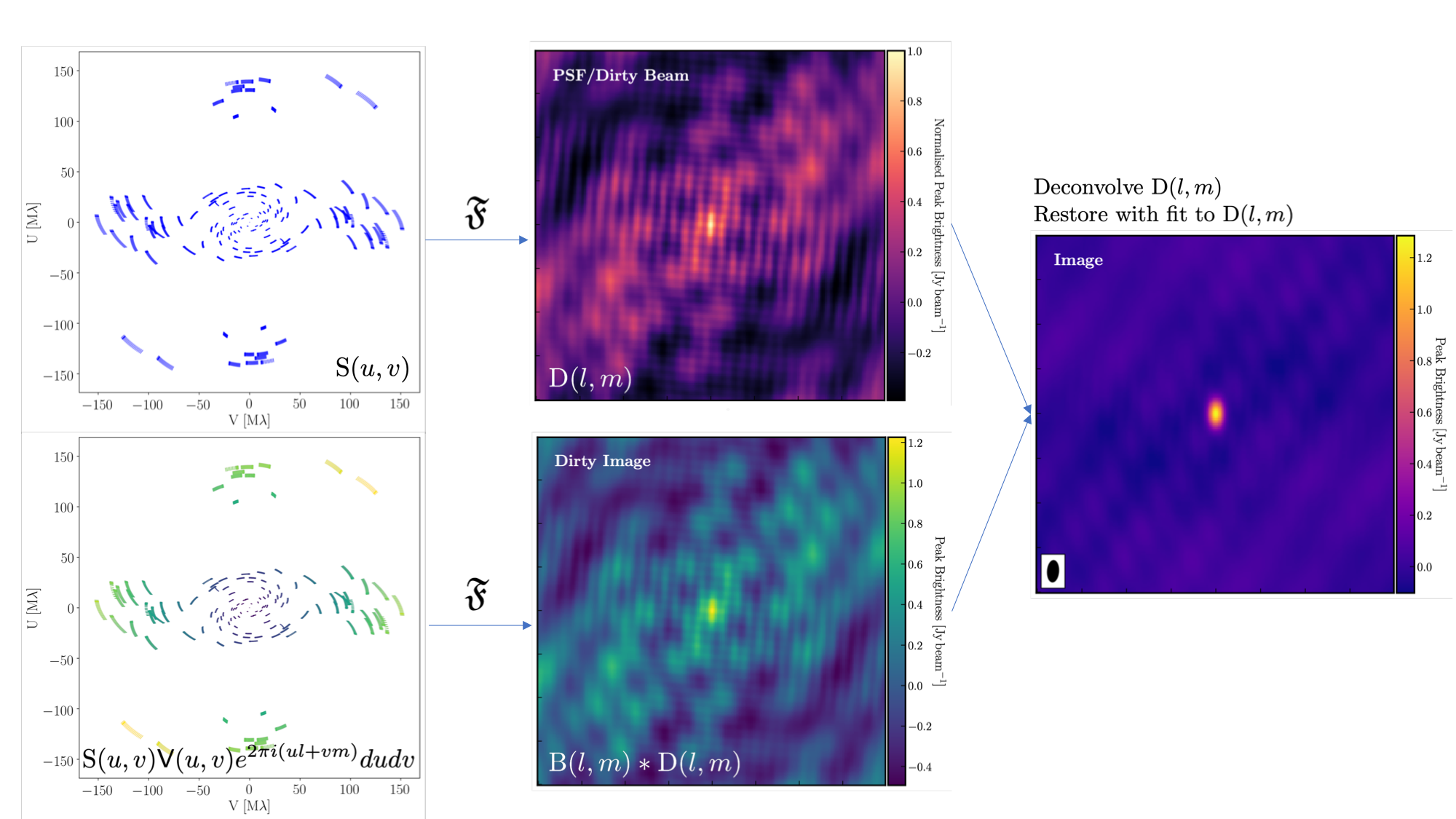The figure above summarises the imaging process (using our phase calibrator as an example) and we shall go through this in the following section. If you remember from the lectures, we can represent the visibilities measured by an interferometer, $\mathsf{V} ( u , v )$, with the following equation: $$\mathsf{V} ( u , v ) \approx \iint _ { l m } \mathsf { B } ( l , m ) e ^ { - 2 \pi i ( u l + v m ) } \mathrm{d}l \mathrm{d}m$$ where $\mathsf { B } ( l , m )$ is the sky brightness distribution, $l,m$ are directional cosines and $u,v$ are the coordinates (defined as perpendicular to the source). From the lectures, you will remember that a key factor in interferometric observations is that we have an unfilled uv-plane i.e. we are not sensitive to all spatial frequencies. This makes imaging a fundamentally more difficult problem because we have to deal with arrays with 'holes' in them (also known as sparse arrays). This makes directly Fourier transforming the visibilities nigh-on impossible.

To get around this, we can define a sampling function, $\mathsf{S}(u,v)$, which is equal to 1 where there is a measurement on the $uv$ plane and 0 otherwise. The sampling function is easy to determine because we know exactly where our antennas are at all times, so we therefore know where on the $uv$ plane they will occupy. A good way to visualise $uv$ plane is to imagine the apparent movement of the antennas across the course of the observations as if you were looking at the Earth from the location of the source.

If we multiply each side of the imaging equation by the inverse Fourier Transform of the sampling function, we can Fourier Transform ($\mathfrak{F}$) the imaging equation to give: $$\mathsf{ B } ( l , m ) * \mathsf{ D } ( l , m ) \approx \iint _ { uv } \mathsf{S} ( u , v ) \mathsf{V}( u , v ) e ^ { 2 \pi i ( u l + v m ) } \mathrm{d}u\mathrm{d}v \\\mathsf{ D } ( l , m ) = \iint _ { u v } \mathsf{S} ( u , v ) e ^ { 2 \pi i ( u l + v m ) } \mathrm{d}u \mathrm{d}v$$ where $\ast$ is the convolution operator. You can see that now we can recover the intrinsic source brightness distribution $\mathsf{B}(l,m)$ if we can deconvolve the $\mathsf{D}(l,m)$ term from it. This $\mathsf{D}(l,m)$ term is known as the dirty beam or point spread function (PSF). Luckily this can be derived easily because we know exactly what $\mathsf{S}(u,v)$ is.

Sounds simple eh? Not so! The next step, namely the deconvolution of the PSF from the dirty image, is a little more complicated than it sounds. The deconvolution process is called an 'ill-posed' problem in mathematics. What this means is that, without some assumptions, solutions to deconvolution are often not unique i.e. there are many variants of $\mathsf{B}(l,m)$ that would satisfy the equation. In addition to this, the algorithms used to perform deconvolution can be highly non-linear and can diverge from 'good' solutions.

To help the deconvolution process, we can apply a range of assumptions in order to guide the deconvolution process towards to the most likely sky brightness distribution. These assumptions are often physically based, e.g. the assumption that $\mathsf{B}(l,m)$ is always positive, but the assumptions can vary slightly with each different deconvolution algorithm.

The most commonly used string of algorithms are the CLEAN variants which we shall discuss here. The standard CLEAN algorithm (either Hogbom or Clark) assumes that the sky is sparse, i.e. radio sources are far from each other and that the sky brightness distribution can be represented by point sources (i.e. delta functions). The CLEAN algorithm goes through many cycles in which it identifies the brightest pixels and then removes 10% (the default value) of the flux density at each of these pixels and records the value and position in a model image. CLEAN then calculates the contribution of these removed pixels convolved with the PSF and subtracts it from the dirty image. This is known as a 'minor cycle'.

After a certain number of minor cycles, the model is the Fourier transformed and subtracted from the visibility data, which are then re-Fourier transformed back to make a new dirty image, which will have less bright emission and fewer contributions from the dirty beam. This is known as a 'major cycle'. These cycles are continued until the final dirty image (or the 'residual image') is indistinguishable from noise. The following gif shows the CLEAN process on the 1848+283 phase calibrator we are about to image. You can see how the model gets built up and the contribution from the PSF reduces over each major cycle and each CLEAN iteration (which removes the 10% from the brightest pixel).Once deconvolution is complete and the residual image looks close to noise, the model is convolved with the synthesised beam and added (without all the horrible sidelobes) back into the data. The synthesised or restoring beam is an idealised Gaussian fit to the PSF and can be thought of as the effective resolution of the interferometer array. The final image is shown in the right most panel of the figure at the start of this section. The synthesised beam size is represented by the elliptical overlay in the bottom left of the figure.

3B. Determining the imaging parameters

Let's get on with making our first image using the CASA task tclean. In the CASA prompt, type default(tclean) and then inp. There is a quite a few inputs which can be quite daunting, however, this task is very versatile and conducts imaging for many different observing set-ups (e.g. spectral line, wide-field etc.). If you wonder what is best for your data, consult the CASA docs.

There are however some things that we always need to decide and set when imaging which are:

1. imagename - this is self explanatory, you need to set an image name which should describe the imaging step and object imaged.

2. field - this is the name of the field that you want to observe and again is self-explanatory.

3. cell - this is the angular size of each pixel in our output image. To estimate this, we need to determine the resolution of our interferometer. We can do this by looking at the $uv$ distance (i.e. the radial distance of measurements in the $uv$ plane). In CASA type the following commands:

default(plotms)
vis='1848+283_J1849+3024.ms'
xaxis='uvwave'
yaxis='amp'
field='1848+283'
antenna="*&*"
spw='7'
correlation='RR'
plotms()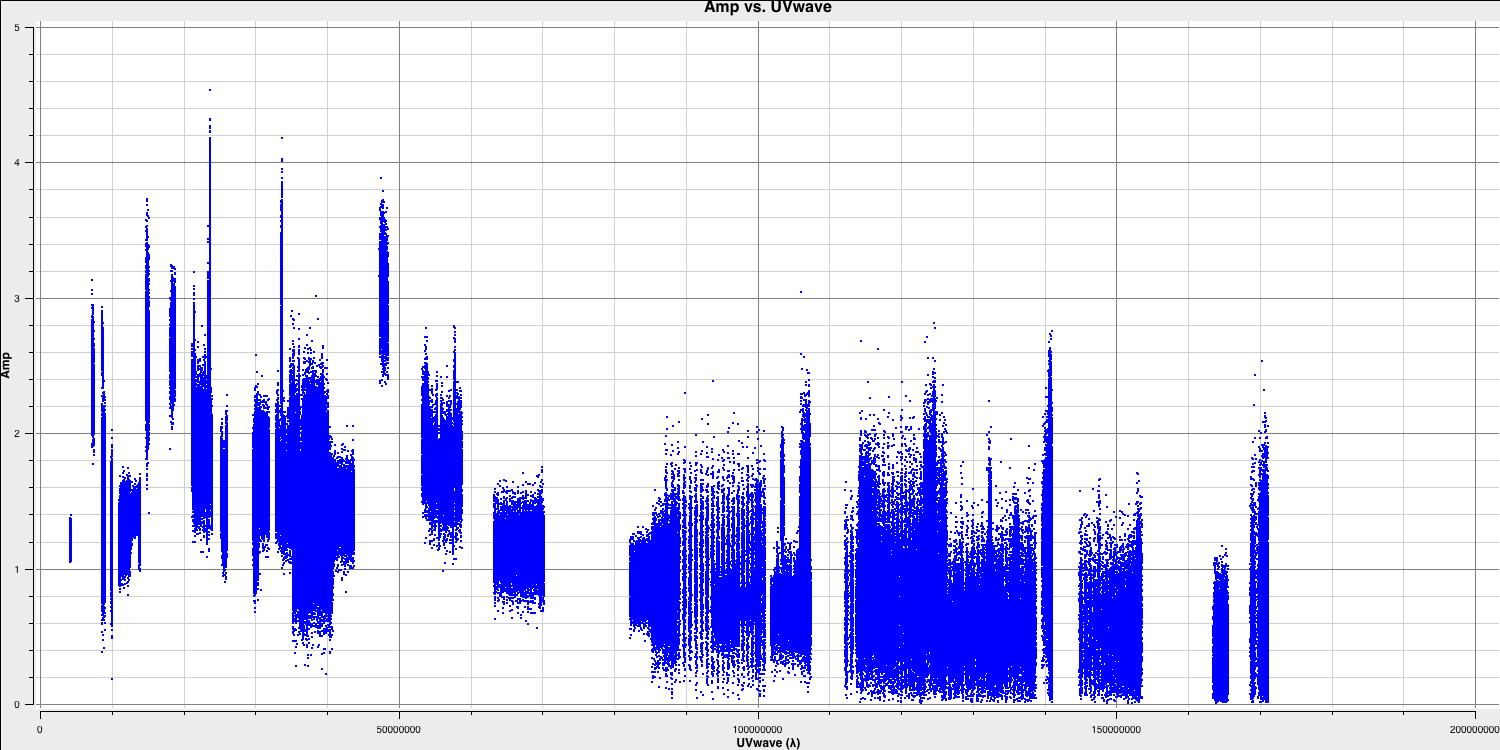Only the highest frequency spectral window is chosen (as this corresponds to the best resolution, remember $\lambda/B$), and only one correlation is picked. These are picked to improve the plotting time.

In this plot, the greatest projected baseline length of the observation which corresponds to the highest possible resolution. Note that we have used some other parameters in plotms to plot grids etc.

To obtain a good value for cell, you should read the highest $uv$ distance value (which is approximately $1.7\times10^{8}\,\lambda$). With this obtained we can convert the values into a resolution (remember the resolution of an interferometer is $\sim\lambda/D$ and the $\lambda$s will cancel out!).

There is one other factor that we have missed out and that is Nyquist sampling ($N_\mathrm{s}$). Remember that, during imaging, we have to fit a 2D Gaussian to the PSF in order to get our synthesised/restoring beam. The minimum number of pixels required to obtain a fit to a Gaussian must be at least 3 pixels but often (especially for VLBI where the PSFs are highly non-Gaussian) more pixels are used.

Based upon the aforementioned points, an estimate for a good cell size is given by the following equation: $$\mathrm{cell} \approx \frac{180}{\pi N_\mathrm{s}} \times \frac{1}{D_\mathrm{max}\,\mathrm{[\lambda]}}~\mathrm{[deg]}$$

In this case assuming a $D_\mathrm{max} = 1.7\times10^{8}\,\lambda$ and $N_\mathrm{s}$ of 5 pixels, you should obtain a cellsize of around $0.24\,\mathrm{mas}$ i.e. cell=['0.24mas'].

4. imsize - This describes the number of pixels your image is going to be on a side. This is often a compromise between computing speed and deconvolution performance. You want an image which is not too large, but large enough that you can remove the PSF sidelobes (see the imaging figure above!). If your source is extended, then the image size may also need to be larger to account for this. On top of this, we also want to set an image size which is optimal for the underlying algorithms in tclean. According to CASA, a good number to choose is even and divisible by 2, 3, 5 and 7 only!. An easy rule of thumb is to use an image size of $2^{n}\times 10$, where $n$ is an integer, such as 80, 160, 320, 640, 1280, 2560 or 5120. In this case, we are going to adopt an image size of 640 i.e. imsize=[640,640]

5. deconvolver - The choice of deconvolution algorithm is highly dependent upon your source and your interferometer. To establish the correct choice for you data consult the CASA docs. A good rule of thumb of what to pick is the following:

• Do you expect that your sources are extended i.e. $\gg$ than the resolution? - consider multiscale (this deconvolution algorithm, assumes the sky is comprised of many Gaussians which is better for modelling fluffy emission compared to using delta functions)
• Does your observation have a large fractional bandwidth i.e. a good rule of thumb is $\mathrm{total~bandwidth/central~frequency} = \mathrm{BW}/\nu_c > 10\%$? - consider mtmfs (this models the change in flux/morphology of the source across the bandwidth and failures to model this can cause un-deconvolvable errors in the image)
If the source is extended too, implement multiscale deconvolution by setting the scales parameter.
• Do you expect that the source has polarisation structure? - consider clarkstokes (which cleans the separate polarisations separately)
• If not, then we would expect we have a small bandwidth and compact structure therefore the clark algorithm should be sufficient.

In our case, our phase calibrator (by definition!) should be compact, we have a small fractional bandwidth ($\mathrm{BW}/\nu_c \sim 0.128\,\mathrm{GHz}/5\,\mathrm{GHz} \sim \mathbf{2.6\%}$), and we don't care about polarisation here. This means that setting deconvolver='clark' should be fine!

6. niter - following on from the deconvolver, this parameter determines the number of iterations the deconvolution algorithm is going to use. If you remember earlier, for each iteration a percentage of the flux of the brightest pixel is removed from the dirty image therefore the larger the niter, the more flux is removed. Luckily we are going to do this interactively and inspect the residuals after each major cycle so we can adjust niter on the fly. For this, we won't go so deep therefore we set niter=1000 and we will do it interactively so set interactive=True.

7. weighting - the weighting parameter determines how the various baselines are represented in the imaging routine. Uniform weighting makes each baseline in the $uv$ plane have equal weight, which typically gives images with the highest resolution, whilst natural weighting maximises sensitivity and increases the contributions from data points/baselines which are closer in the $uv$ plane. For this imaging, we shall run the default of weighting='natural'. We shall investigate the effects of changing the weighting in the 3C277.1 data reduction school and part 3 of this workshop.

3C. Inputting the parameters and executing tclean

So now that we have decided what parameters should be set and what values should be used, let's input these into our NME_J1849_skeleton.py script.

• Go to step 1 in NME_J1849_skeleton.py and you should find the following:

### 1) Image phase calibrator
mystep = 1

if(mystep in thesteps):
print('Step ', mystep, step_title[mystep])

# Image the phase calibrator with tclean

os.system('rm -r '+phscal+'_p0.*')
tclean(vis=phscal+'_'+target+'.ms',
imagename=phscal+'_p0',
stokes='pseudoI',
psfcutoff=0.5,
field='xxx',
imsize=['xxx','xxx'],
cell=['xxx'],
gridder='xxx',
deconvolver='xxx',
niter='xxx',
interactive=True)
• Replace the 'xxx' with the imaging parameters that we have just derived. Remember to check tclean (using inp tclean and help tclean) to ensure that the variables included are the right format. e.g. imsize=[<integer>,<integer>] and not strings!

• Some final comments on other parameters. The use of stokes='pseudoI' is to ensure that the telescope with a single observed polarisation (SV) is included when imaging (to improve sensitivity). To generate true stokes I images, we would need to combine both RR and LL ($I = (RR + LL)/2$). The pseudoI just assumes that there's equal power on the other polarisation which was not observed. While this is ok for non-polarimetric imaging like this case, we would not want to use pseudoI for polarisation studies. Secondly, the use of psfcutoff=0.5 is to ensure that the highly irregular psf is fitted properly.
• Execute this block of code in the CASA prompt using the syntax we introduced earlier i.e.

## In the CASA prompt
runsteps = 
execfile('NME_J1849_skeleton.py')
• If you are having errors, compare your input to step 1 in NME_J1849.py to see where you went wrong. Often it's just due to a missing comma or incorrect indenting.

3D. CLEANing in tclean

Now that you have executed tclean, you should see a flurry of activity in the logger. The algorithm will grid the visibilities with the appropriate weights and produce the PSF, ready for deconvolution and inversion of the visibilities to make our dirty image. We can guide the deconvolution process so that it doesn't diverge (as explained in part A of this section).

We guidance comes via the use of masks. These masks tells CLEAN which pixels should be searched for flux to be removed and the PSF deconvolved. These masks should be set in regions which you think contains real flux. These typically look like the PSF shape in the case of point sources (see part A of this section for the PSF shape). Because we set interactive=True, CASA returns a GUI similar to that shown below where we can set masks and guide the deconvolution process.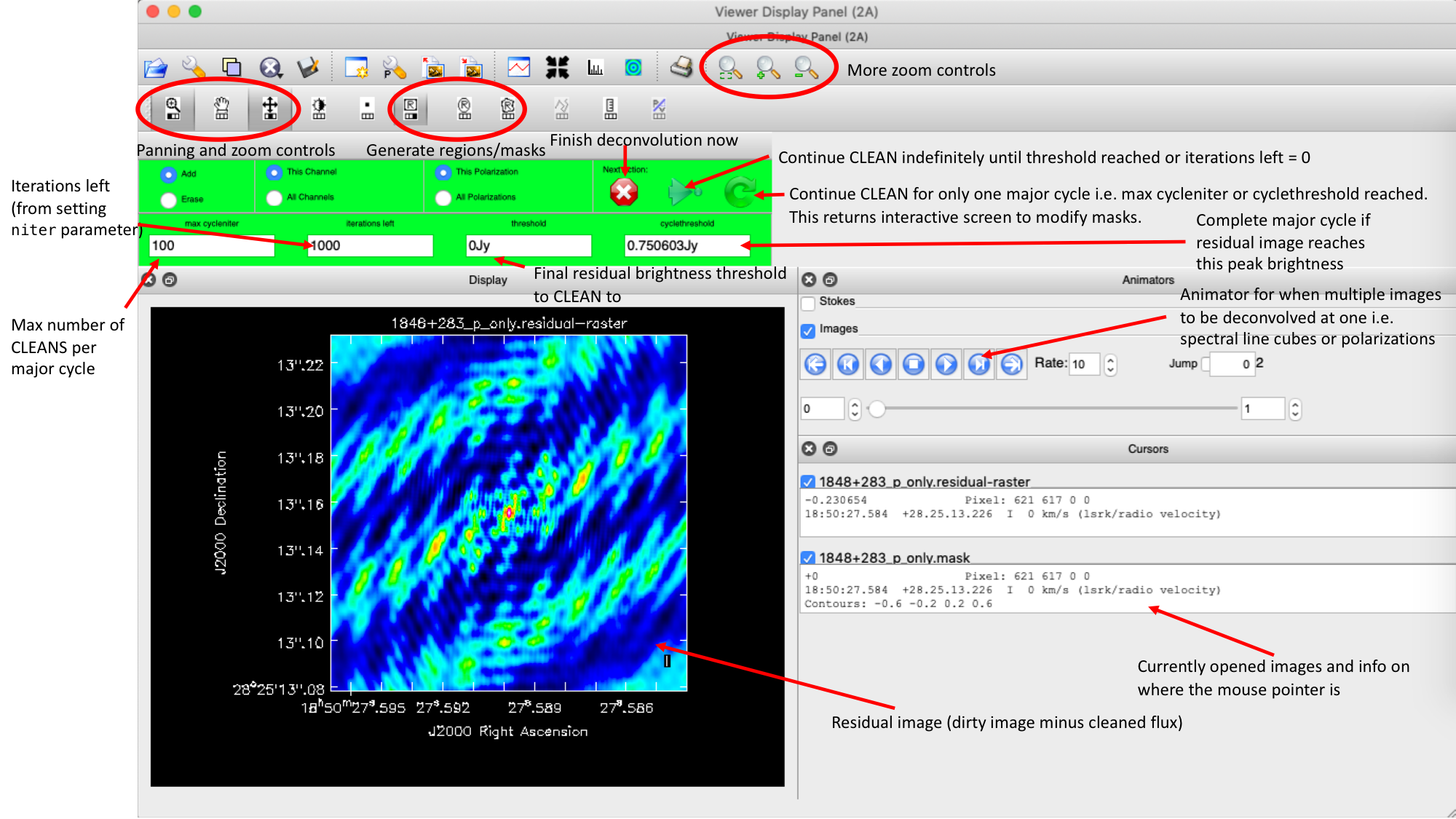Here we can see the dirty image. At the centre of the image, there is a bright source with the characteristic PSF shape around it. It is here that we want to set our mask.

• To set the mask we want to use the region buttons (e.g.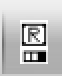). Note that the filled rectangle of the three rectangles on these buttons, corresponds to the mouse buttons. These can be adjusted and re-assigned to different mouse buttons by clicking on them.
• The gif below shows how we can set the mask and run the next major cycle. Important Note that to set the mask, we need to double-click with the appropriate button (in this case, the right mouse button) and the box should change from green to white.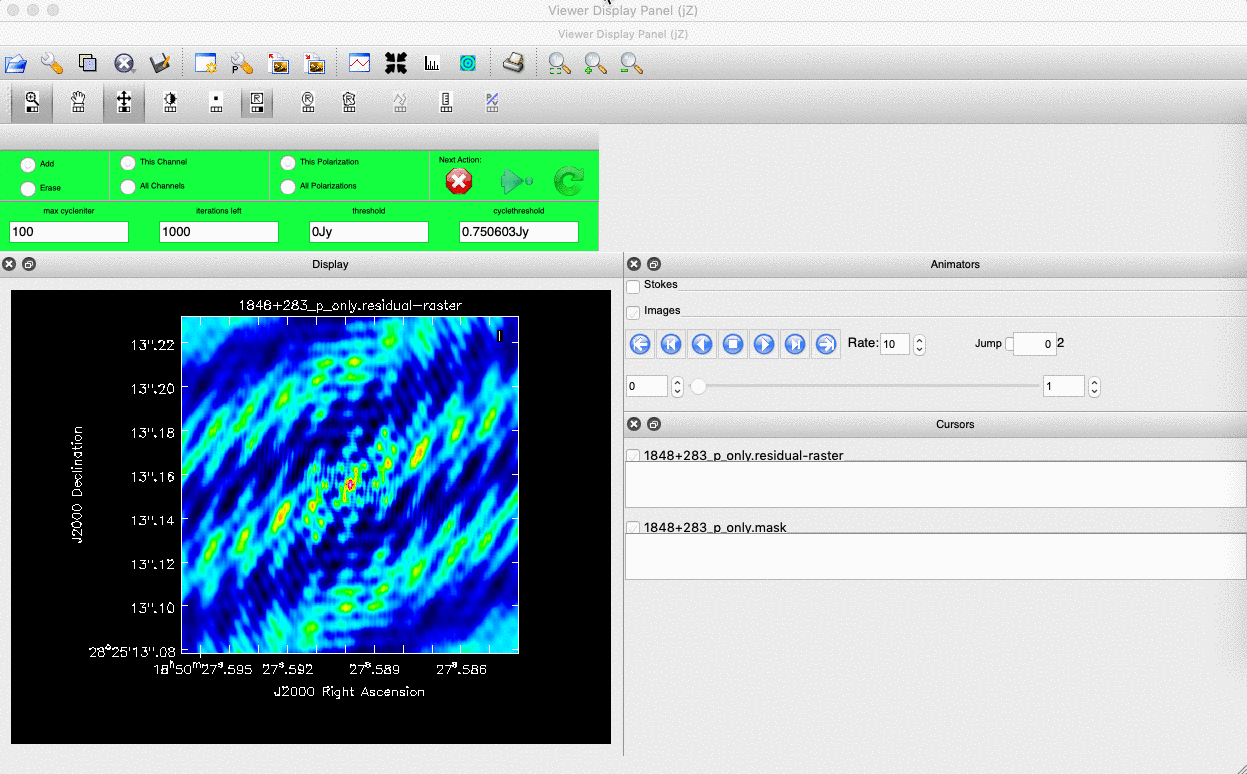• Once the green arrow button has been pressed, the next major cycle will run and the GUI will freeze. This process will remove some flux, deconvolve the PSF and begin the process of generating the model as explained in part A. Once these contributions have been removed from the visibilities, the task will generate another residual image with less bright flux and re-open imview.
• Continue with the CLEANing process and modify the mask as appropriate. Note that you can delete parts of the mask by clicking on the erase button, overlaying the green box on the region to remove and double clicking to remove that portion.
• Over the course of the CLEAN process you should see the PSF imprint getting removed and the residual turning into noise (or noise + calibration errors). Below we show the gif again from part A to show what you should see at the end of each major cycle in your imview GUI.• After around 3 major cycles, you should see some low flux density structure appear which shows that our phase calibrator is not a true point source so the assumption from part 1 is slightly wrong. We will use the model generated with CLEAN to correct for this soon!
• Continue until around major cycle 6, where the source is indistinguishable from noise, and click on the red cross to stop cleaning.

With CLEAN stopped and completed, the algorithm shall take the model image (the delta functions) convolve it with the fitted PSF i.e. synthesised beam and add this onto the residual image to generate the image. Let's have a look at what clean has made:

• Type !ls into the CASA prompt.
• You should see some new files which are CASA images. These are:
• 1848+283_p0.image - The model convolved with the synthesised beam added into the residual image
• 1848+283_p0.residual - The noise image after CLEAN deconvolution (seen in the interactive prompt during CLEANing)
• 1848+283_p0.model - The underlying estimated sky brightness model by CLEAN
• 1848+283_p0.psf - The FT of the sampling function that is deconvolved
• 1848+283_p0.pb - The primary beam response across the image (Note this is not correct for heterogeneous arrays yet)
• 1848+283_p0.sumwt - The sum of weights (not needed)
• Type imview into the CASA prompt and open these images. Try to discern where these images originate from by comparing it to the imaging 101 we presented in part A.
• Look at the 1848+283_p0.image. The source is fairly point-like but the background noise looks very non-gaussian i.e. there's stripes and negatives in a non-random way. This is a tell-tale sign of calibration errors. This is expected as we have not corrected for any amplitude errors nor have we accounted for the slight non-point like nature of our calibrator.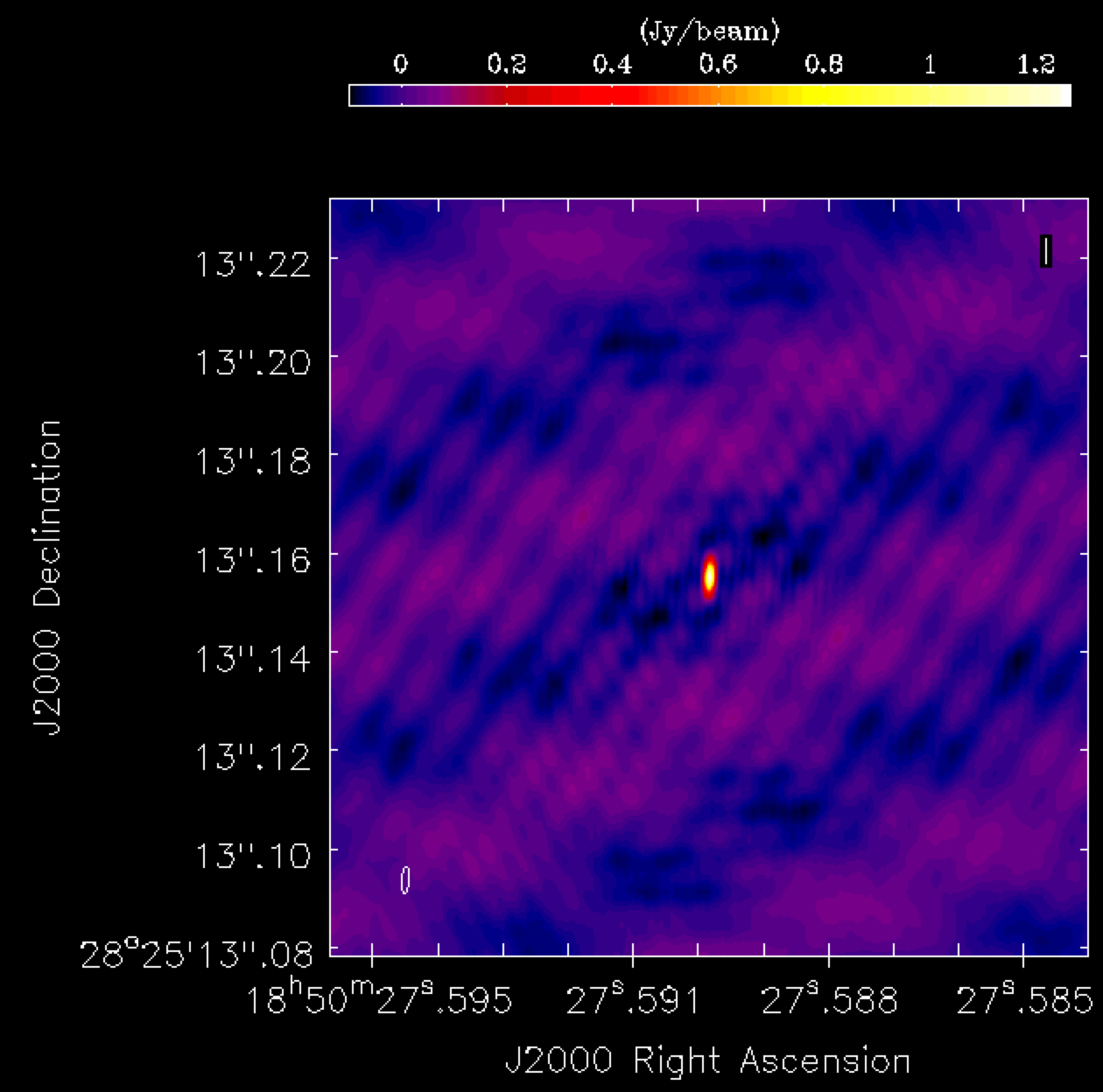• Select the 1848+283_p0.model, they can use 'marker' to view it and the 'spanner' to change the x and y increments to view all the model components.

3E. Tracking calibration improvements

One key thing we should do whenever we attempt to improve calibration is to track the improvements in the image fidelity. We can do this by tracking the peak brightness ($S_p$), the r.m.s. noise ($\sigma$) and also the signal-to-noise ratio ($\mathrm{S/N} = S_p/\sigma$).

Calibration errors typically cause flux to be dispersed into the noise thus increasing it, and reducing the S/N. We can gauge whether our calibration (to be performed in the next steps) is good by tracking the improvement in S/N. An increasing S/N shows that we are bringing back the mis-calibrated flux from the noise to the source. The peak and r.m.s. values are good for seeing if the amplitude calibration works. Bad amplitude calibration can often change the flux scaling so the rms and peak both change in one direction (which is wrong!).

To get these values, we need to use the task imstat which derives statistics from the image. To get the peak and rms we need to define two boxes. The first should be away from the source in an 'empty' area to get the rms, while the second should be over the source in order to extract the peak pixel value.

• Look at step 2 in the script.

### 2) Get image information
mystep = 2

if(mystep in thesteps):
print('Step ', mystep, step_title[mystep])

# Measure the signal-to-noise
rms=imstat(imagename='%s_p0.image'%phscal,
box='xxx,xxx,xxx,xxx')['rms']
peak=imstat(imagename='%s_p0.image'%phscal,
box='xxx,xxx,xxx,xxx')['max']

print('Peak %6.3f, rms %6.3f, S/N %6.0f' % (peak, rms, peak/rms))

• You can see that we need to set the two boxes which are defined by their bottom left corner (blc) and top right corner (trc) coordinates. The box parameter has the syntax box='blcx,blcy,trcx,trcy'. Use the imview to determine these boxes.
• If you are happy with your boxes then run step 2.

The plot below illustrates an example of suitable boxes for an image size of 640x640 pixels and 0.24 mas cell size. These boxes produced a peak brightness, $S_p = 1.269\,\mathrm{Jy\,beam^{-1}}$, a rms noise of $\sigma = 0.033\,\mathrm{Jy\,beam^{-1}}$ and so a signal-to-noise, $\mathrm{S/N} \sim 38$. You should have values close to this. If you do not, then you may not have cleaned deeply enough.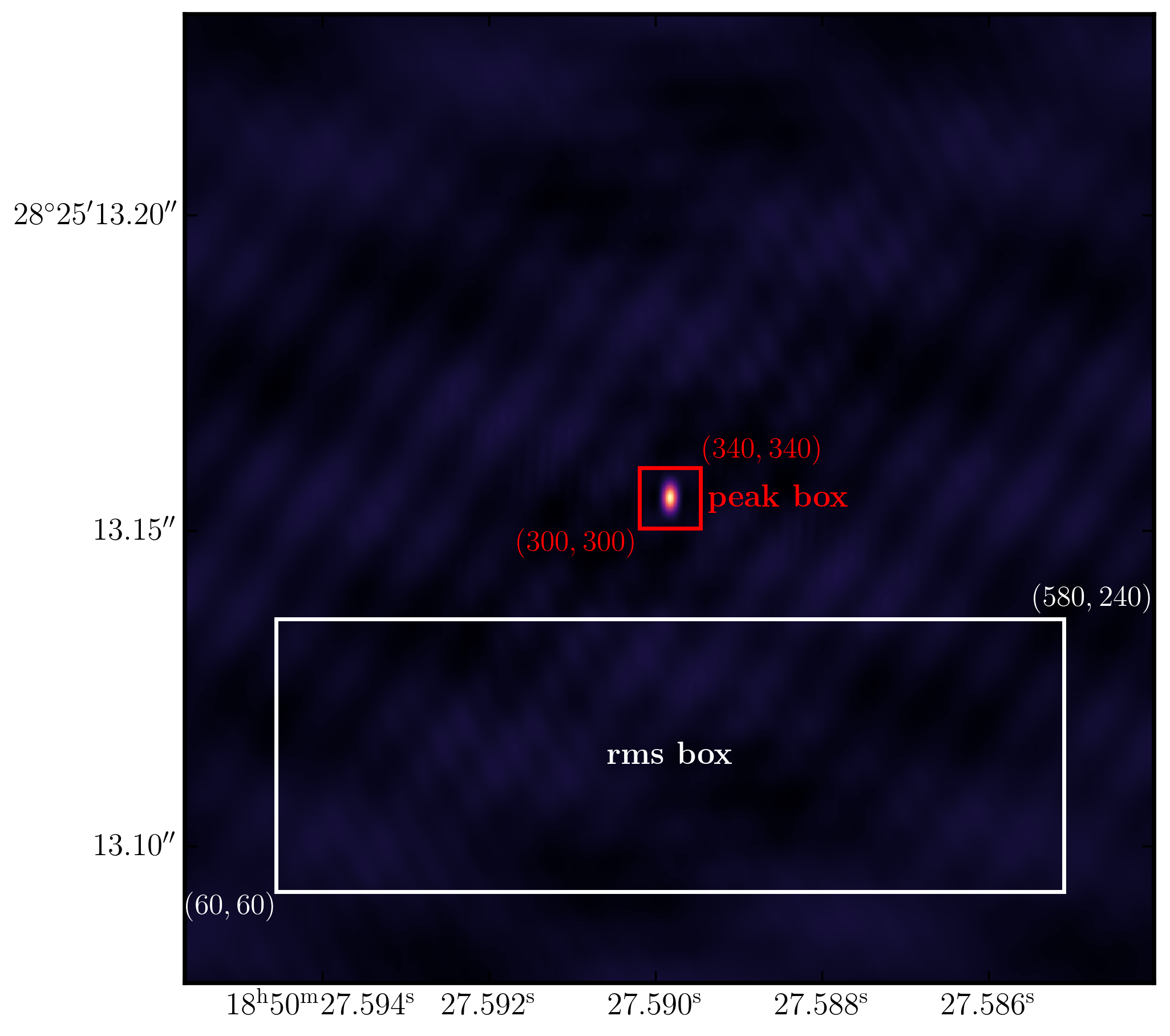4. Correct residual delay & phase errors

Fringe-fitting should have fixed our delays and phases to the first order and often this is sufficient for phase referencing. However, as we had seen at the end of part 1, there are some baselines (mainly to SH/Shenshen) whose delay solutions are unstable. Normally, this indicates that the instrumental delay changes over the course of the observation which may be induced by receiver/correlator problems. If the phase calibrator is not that bright (as is often the case), then we cannot correct for this as we cannot obtain a delay solution for each spw separately. However, in this case, the phase calibrator is bright enough so we can do this.

Secondly, in VLBI observations, the phase calibrator is often not a point source, therefore we need to derive phase corrections that are only representative of the atmospheric phase shifts and not any source structure (which causes non zero phases). This means that the solutions transferred to the target are representative of just the atmosphere. We have seen that this is the case for our phase calibrator which is not quite a true point source, therefore we should derive corrections that take this into account. To do this, we need to use a model rather than our initial point source assumption that was used when fringe-fitting.

4A. Generating the model visibilities

To generate our model for calibration, we use the task ft. This task takes the CLEAN model and Fourier transforms it into the measurement set under the $\texttt{MODEL}$ data column. CASA uses this to derive the corrections by comparing the actual visibilities with the model visibilities rather than using a model of a point source.

• Have a look at step 3 and you can see the task ft
### 3) Generate model column for self-cal
mystep = 3

if(mystep in thesteps):
print('Step ', mystep, step_title[mystep])

# Fourier transform model into the visibilities
ft(vis='%s_%s.ms'% (phscal,target),
field='xxx',
model='xxx',
usescratch=True)
• Replace the field with the phase calibrator name and the model with the model image. The usescratch is set to True to generate a physical $\texttt{MODEL}$ column which speeds up calibration but uses up disk space!
• With all these set, execute step 3!

Let's have a look at the visibilities and the model visibilities which will be used to derive solutions.

• In the interactive CASA prompt (not the script!), use plotms plot amplitude versus uv distance (in wavelength) for all baselines to Effelsberg. Use just one correlation and/or one spw if it takes a long time to load the plot
• Change the column in the plotms GUI to the model column and the data_vector-model columns (go the the axes tab and use the column drop down menu!) to see the differences between the real and model visibilities.
• Repeat for the phases

In the plot below, python has been used to over-plot the real and model visibilities for both amplitude and phase versus uvwave (rr correlation only and all baselines to EF). The bottom panel is the scalar subtraction of the data-model visibilities.As you can see, there are large discrepancies in the amplitude vs uvwave plots. This is expected as the initial fringe fitting in part 1 only corrects for the phases and not the amplitudes. Therefore, the phases are generally good compared to the model apart from the baseline to Shenzhen ($\sim 120\,\mathrm{M\lambda}$), which have some residual delay errors that cause the phases to deviate, as we discussed earlier and which we shall correct for now!

4B. Refining the delays

• Take a look at step 4 in your skeleton file. You should see that we are going to use gaincal to derive our delay corrections. This multi purpose task can be used in a miriad of ways to calibrate your data.

### 4) Refine delay calibration
mystep = 4

if(mystep in thesteps):
print('Step ', mystep, step_title[mystep])

# Gain calibration of phases only
os.system('rm -r '+phscal+'_'+target+'.K')
gaincal(vis=phscal+'_'+target+'.ms',
field='xxx',
caltable=phscal+'_'+target+'.K',
corrdepflags=True,
solint='xxx',
refant='xxx',
minblperant='xxx',
gaintype='xxx',
parang='xxx')
plotms(vis=phscal+'_'+target+'.K',
gridrows=2,
gridcols=3,
yaxis='xxx',
xaxis='xxx',
iteraxis='xxx',
coloraxis='xxx')

We need to set some parameters here and the most important is the solution interval. In this case, our phase calibrator is bright enough to get a solution interval per scan and per spw whereas in fringe-fitting we combined the spws. The delays on Shenzhen changes across the spw and time (which is not normal!) so we can use gaincal to correct for this. We want to maximise S/N, therefore our solution interval should be the whole scan i.e. solint='inf'.

With regards to the other parameters, here are some hints to help you fill them some of them in.

• refant - remember how we decided what the reference antenna should be in part 1!
• gaintype - there is a specific string to do delay calibration, use the help command to find it
• parang - in part 1, we applied the parallactic angle correction on the fly, however, we applied this correction to the data when we split out the corrected data! Do we need to apply it twice?

Remember to also fill in the plotms task too. If you have done that execute step 4 and you should get solutions like this:For most telescopes, the delays are near zero hence the fringe-fitting solutions were good. We can see for Shenshen that there are much larger corrections which vary across the spw and polarisations. These solutions look good and should correct for our delay issues. Let's use these solutions and now refine the phases.

4C. Refining the phases

Using these new delay solutions, we should now be able to derive corrections to the phases against time. Again we need to derive a solution interval!

• Using plotms, type the following into your CASA prompt:

default(plotms)
vis='1848+283_J1849+3024.ms'
xaxis='time'
yaxis='phase'
spw='1'
field='1848+283'
correlation='RR,LL'
avgchannel='32'
antenna='EF&*'
iteraxis='baseline'
plotms()

You should see that the phases are close to zero for most baselines, however some still have large deviations (e.g. Shenshen, see plot below). We ideally want a solution interval that gives us at least two data points per scan so that the linear interpolation can track effectively between scans and we can apply more accurate phase solutions to the target field. A 20s solution interval can give us three solutions with enough S/N to get good solutions!Note that the plot above shows all spectral windows which are colorised by spw.

• With this in mind, look at step 5 in the skeleton script

### 5) Refine phase calibration
mystep = 5

if(mystep in thesteps):
print('Step ', mystep, step_title[mystep])

# Gain calibration of phases only
os.system('rm -r '+phscal+'_'+target+'.p')
gaincal(vis=phscal+'_'+target+'.ms',
field=phscal,
caltable=phscal+'_'+target+'.p',
corrdepflags=True,
solint='xxx',
refant='xxx',
gaintype='xxx',
minblperant='xxx',
calmode='xxx',
gaintable=['xxx'],
parang='xxx')
plotms(vis=phscal+'_'+target+'.p',
gridrows=2,
gridcols=3,
plotrange=[0,0,-180,180],
yaxis='xxx',
xaxis='xxx',
iteraxis='xxx',
coloraxis='xxx')

As with the delay calibration, many of these should stay the same but here are some more hints for the new parameters of parameters that should be changed from the delay calibration introduced here. Note that we set the yaxis plotrange to be -180 to 180 degrees so we can see the scale of the phase corrections.

• gaintype - again, check the help docs to find the right code for gain / phase calibration (not delays!)
• calmode - use the help docs to find the code to do phase only calibration!
• gaintable - set this so that the delay solutions are applied on the fly
• minblperant - we want to make closure quantities so that baseline-based errors are not corrected for (the visibilities will be too constrained by the model!). Therefore for phases we need 3 baselines per antenna and 4 for amplitudes.

• If you are happy, execute step 5 and you should see the following phase solutionsYou can see that for most baselines the corrections are small, however, for the Shenshen telescope, these corrections are quite large. To see if these corrections improve our data, then we need to apply them to these data.

4D. Apply corrections to phase cal

Let's apply the solutions using applycal

• Take a look at step 6 and the inputs to the task applycal

# 6) Apply delay and phases
mystep = 6

if(mystep in thesteps):
print('Step ', mystep, step_title[mystep])

# Apply these solutions to the phase cal
applycal(vis='%s_%s.ms'% (phscal,target),
field='xxx',
gaintable=['xxx','xxx'],
gainfield=['xxx','xxx'],
interp=['xx','xx'],
applymode='xxx',
parang=False)

For this application of the solutions, we just want to calibrate the phase calibrator in order to inspect how well the solutions improve the visibilities. Because of this, the interpolation mode is not too important i.e. nearest or linear is identical here. As only a few solutions fail, the flagging applymode is ok here!

• Set the parameters of step 6 and execute the task
• Once complete, re-plot the plotms command from part C. of this section to see the improvements. Remember to set ydatacolumn='corrected'

The plot below shows the improvement on the EF to SH baseline with these new delay and phase solutions applied. There are some errant data which could be flagged, however this will not affect the final result significantly!.If you wish to flag the data, use the interactive flag tool and iterate over the SH baselines, flagging as you go along (use the previous plotms commands but set spw='' and antenna='SH&*')

5. Correct amplitude errors on phase-cal

5A. Re-image phase calibrator

With the corrections applied, we should image the phase calibrator again to generate an improved model for the next round of calibration.

• Take a look at step 7 in the skeleton file. You can see that we have now combined the deconvolution, the generation of the MODEL column and the image statistics all in the same step. The parameters you enter should be the same as what has been done before.

### 7) Image phase calibrator again!
mystep = 7

if(mystep in thesteps):
print('Step ', mystep, step_title[mystep])

# Image the phase calibrator with tclean

os.system('rm -r '+phscal+'_p1.*')
tclean(vis=phscal+'_'+target+'.ms',
imagename=phscal+'_p1',
stokes='pseudoI',
psfcutoff=0.5,
field='xxx',
imsize=['xxx','xxx'],
cell=['xxx'],
gridder='xxx',
deconvolver='xxx',
niter='xxx',
interactive=True)

# Measure the signal-to-noise
rms=imstat(imagename=phscal+'_p1.image',
box='xxx,xxx,xxx,xxx')['rms']
peak=imstat(imagename=phscal+'_p1.image',
box='xxx,xxx,xxx,xxx')['max']

print('Peak %6.3f Jy/beam, rms %6.3f mJy/beam, S/N %6.0f' % (peak, rms*1e3, peak/rms))

ft(vis=phscal+'_'+target+'.ms',
field='xxx',
model='xxx',
usescratch=True)

Using the same boxes as in step 2, I got $S_p = 1.274\,\mathrm{Jy\,beam^{-1}}$, $\sigma=0.033\,\mathrm{Jy\,beam^{-1}}$, $\mathrm{S/N}= 39$. The improvement is very very modest because the phases were mostly calibrated by fringe fitting. Only the Shenshen telescope, which was not properly calibrated during fringe fitting, had large corrections applied.

5B. Obtain amplitude solutions

In the next step, we want to use the improved model visibilities to correct for the amplitudes. As was shown in section 2A of this tutorial, where we plotted the amp vs. uv distance plots, there were some mis-scaled amplitudes compared to the model. This step will correct for this.

• Look at step 8

### 8) Derive amplitude solutions
mystep = 8

if(mystep in thesteps):
print('Step ', mystep, step_title[mystep])
os.system('rm -rf'+phscal+'_'+target+'.ap')
gaincal(vis=phscal+'_'+target+'.ms',
caltable=phscal+'_'+target+'.ap',
corrdepflags=True,
field='xxx',
solint='xxx',
refant='xxx',
solnorm='xxx',
gaintype='xxx',
minblperant='xxx',
calmode='xxx',
gaintable=['xxx','xxx'],
gainfield=['xxx','xxx'],
interp=['linear','linear'],
parang=False)
plotms(vis=phscal+'_'+target+'.ap',
gridrows=2,
gridcols=3,
yaxis='xxx',
xaxis='xxx',
iteraxis='antenna',
coloraxis='spw')

While most of these parameters can be the same as the phase only calibration, we need to change a few. Here are some hints for the ones that need to be changed.

• solint - solution interval. Most amplitude errors are due to the instrument, we expect this to vary slower than the phase. In addition, amplitude solutions need higher S/N per solution interval. A good rule of thumb at GHz frequencies is 3 x the phase only solution interval.
• solnorm - this normalises the amplitude solutions so the flux scale remains the same. Seen as we have already set this scale we should set this to True!
• calmode - we want to do an amplitude and phase solution as these are normally more stable. Check the help for the right code
• gaintable and gaintable - remember that any calibration is done on the data column so we need to apply the other calibration tables on the fly!

• If you are happy then put the parameters into both tasks and run step 8.

The plot below, shows the amplitude solutions for each telescope and spw. You can see that for the majority of telescopes, the solutions are close to unity, hence they were well calibrated by the $T_\mathrm{sys}$ measurements we used in part 1. However, some telescopes e.g. Westerbork (Wb) get rescaled by this correction.5C. Apply amp solutions

With the amplitude solutions derived and checked, we should apply them to the phase calibrator so we can investigate the improvements due to this calibration before we apply the solutions to the target source.

• Look at step 9

### 9) Apply all calibration to the phase calibrator
mystep = 9

if(mystep in thesteps):
print('Step ', mystep, step_title[mystep])

applycal(vis='%s_%s.ms'% (phscal,target),
field='xxx',
gaintable=['xxx','xxx','xxx'],
gainfield=['xxx','xxx','xxx'],
interp=['xxx','xxx','xxx'],
parang=False)

• Enter the parameters to apply the solutions to the phase calibrator only
• Run step 9

5D. Re-image phase calibrator with amplitude solutions applied

To ensure that the solutions we have derived are ok to be applied to the target, we should do a final image of the phase calibrator to ensure that the calibration hasn't left many errors (i.e. non-Gaussian noise/stripes/artifacts). This can also flag up some low level RFI as well which will manifest as stripes in the image.

• Look at step 10 and input the parameters to execute tclean again. Note that this time we don't need to use ft to input the model as we should be done with calibration

### 10) Image phase calibrator for last time
mystep = 10

if(mystep in thesteps):
print('Step ', mystep, step_title[mystep])
os.system('rm -r '+phscal+'_ap1.*')
tclean(vis=phscal+'_'+target+'.ms',
imagename=phscal+'_ap1',
stokes='pseudoI',
psfcutoff=0.5,
field='xx',
imsize=['xx','xx'],
cell=['xxx'],
gridder='standard',
deconvolver='xxx',
niter='xxx',
interactive=True)

# Measure the signal to noise
rms=imstat(imagename=phscal+'_ap1.image',
box='xxx,xxx,xxx,xxx')['rms']
peak=imstat(imagename=phscal+'_ap1.image',
box='xxx,xxx,xxx,xxx')['max']

print('Peak %6.3f Jy/beam, rms %6.4f mJy/beam, S/N %6.0f' % (peak, rms*1e3, peak/rms))

When you open the image in imview, you will find that the S/N of the image has dramatically increased. Using the same boxes as before, I managed to get $S_p=1.407\,\mathrm{Jy\,beam^{-1}}$, $\sigma=0.5622\,\mathrm{mJy\,beam^{-1}}$, and so a $\mathrm{S/N}=2502$.

If we look at the amplitude and phase versus uv distance plots again, we can see that the discrepant amplitudes we saw have come back in line and only a very small proportion of data is still mis-calibrated. This bad data shouldn't make much of a difference but you can flag it if you'd like.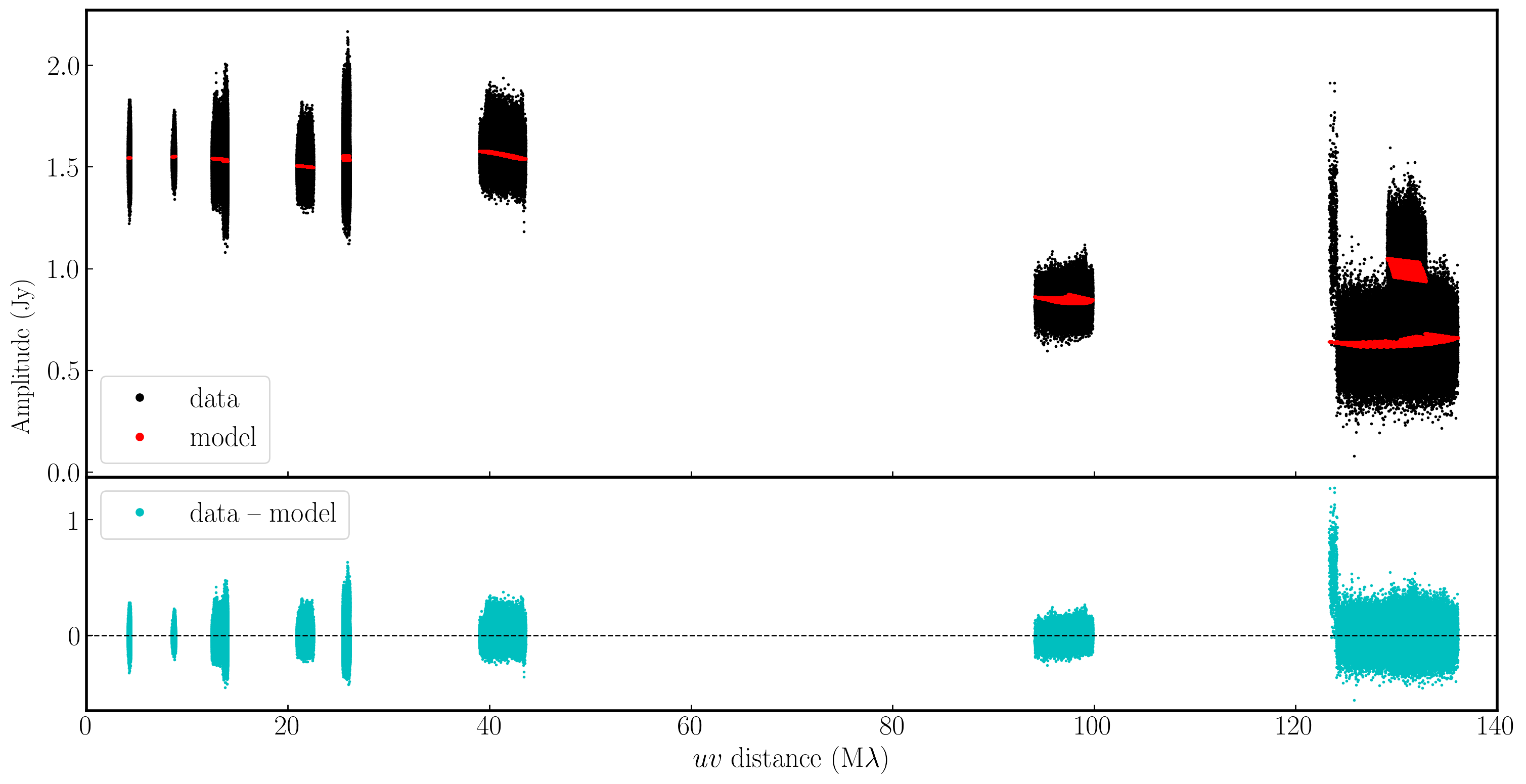5E. Apply solutions to the target

Now that we are broadly happy with the calibration, we should transfer these solutions onto the target source. As the target scans are interleaved between the phase calibrator scans, we should think about the best interpolation scheme for the job. We didn't go into this during part 1 in much detail, but we will do now.

In CASA, there are two main interpolation methods namely nearest and linear. These are simple to understand. Nearest interpolation means that data will be corrected using the nearest data point in the time and frequency space, whereas 'linear' will join a line between the two data points straddling the data you want to correct and use the linear solution at the data location.

If the true solutions do not deviate rapidly or the solutions derived are close to the data you want to correct, then both interpolation schemes are similar. However, when the change is significant, there can be a large difference.

In the plot below, we illustrate a model phase change induced on the signal from a source by the atmosphere over a single antenna. Across the phase calibrator, we assume we track the phase perfectly (red lines), however, we must interpolate across the target scans (with the true phase changes in gray). As you can see, the errors (bottom panel) on the nearest interpolation (which are a step-wise model) are larger than the linear interpolation especially when the phase changes are more severe across the target scan.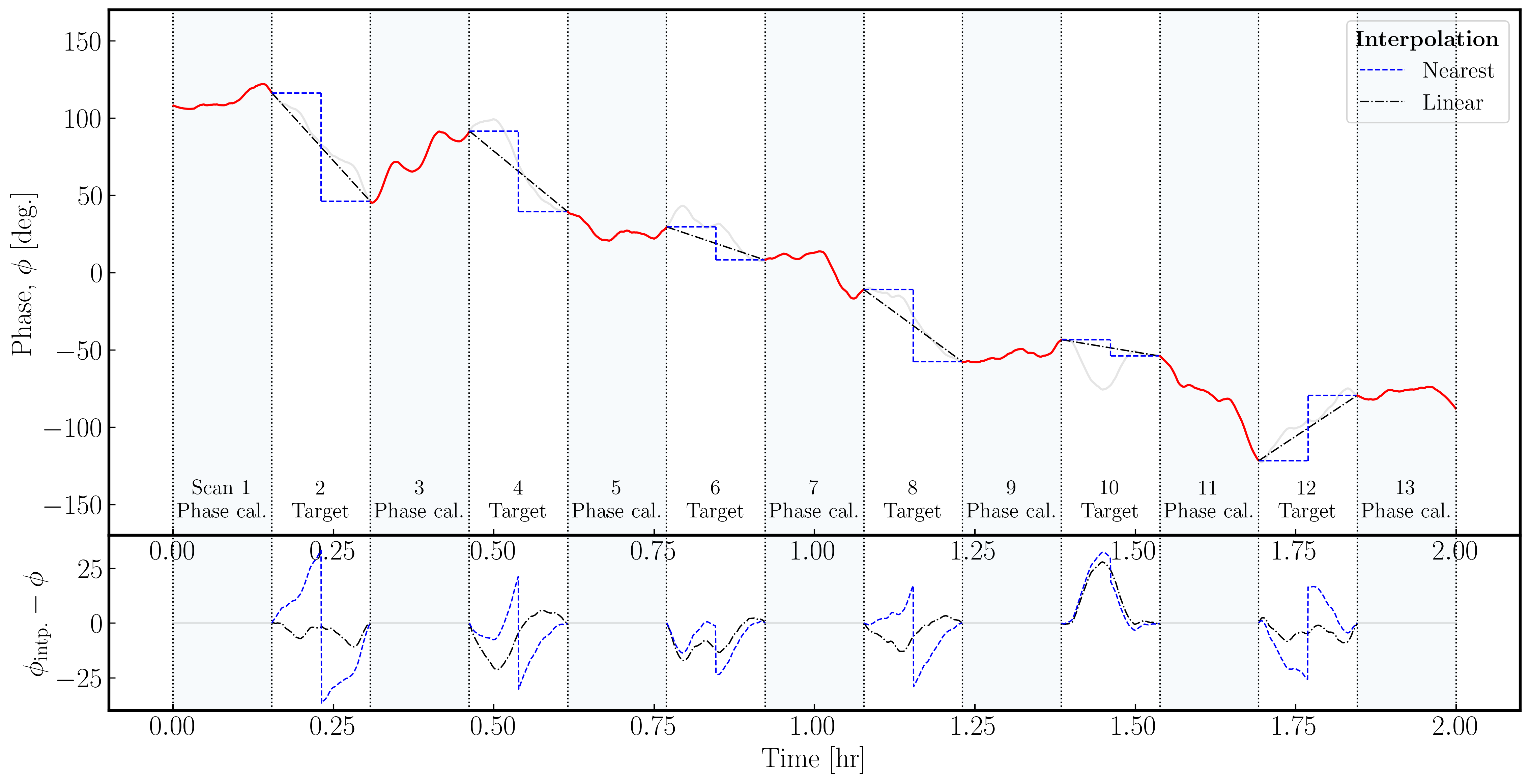It is important to notice that neither interpolation scheme can model any curvature (e.g. scan 10) therefore phase calibrator to target cycle times must be short enough that the phases/amp/delays/rates can be estimated linearly!

Either way, the best scheme here is to use linear interpolation as this generally tracks the target phase better than nearest interpolation. Let's use this knowledge and apply our refined solutions to the target.

• Look at step 11 in the skeleton script

### 11) Apply refined solutions to the target source
mystep = 11

if(mystep in thesteps):
print('Step ', mystep, step_title[mystep])
delmod(vis=phscal+'_'+target+'.ms')
applycal(vis=phscal+'_'+target+'.ms',
field='xxx',
gaintable=['xxx','xxx','xxx'],
gainfield=['xxx','xxx','xxx'],
interp=['xxx','xxx','xxx'],
applymode='calonly',
parang=False)

• Input the parameters to apply all calibration to the target and use linear interpolation to do so. Note that the applymode='calonly' is used to prevent unnecessary flagging of target data.
• Execute step 11

5F. Split target

The final step we need to do involving the phase calibrator is to remove the calibrated target data and put it into a new measurement set. This is to provide an intermediate back-up data set, and so that we can derive some more calibration using just the target source and not the phase calibrator.

• Look at step 12 and input the parameters to split just the target source data into a separate measurement set.

### 12) Split target data out from the measurement set
mystep = 12

if(mystep in thesteps):
print('Step ', mystep, step_title[mystep])
os.system('rm -rf '+target+'.ms')
os.system('rm -rf '+target+'.ms.flagversions')
split(vis=phscal+'_'+target+'.ms',
outputvis=target+'.ms',
field='xxx',
correlation='xx,xx',
datacolumn='xxx')

Because we are not concerned with polarisation in this tutorial, we will only output the parallel hand correlations (LL, RR) to reduce the measurement set size. Also, remember that we want to split out the calibrated data so you need to set the datacolumn parameter.

• If you are happy, then execute step 12 to make the new measurement set called J1849+3024.ms. This is the measurement set we shall work on from now on!

6. Self calibration of target

6A. First target image

With the solutions applied to the target field, we want to assess our target field. Remember that the phase solutions we derived for the phase calibrator will only be approximate because the telescopes look through slightly different patches of sky. This means that we will have gain errors on our target field. In this section, we shall do the same as we did on the phase calibrator and perform self-calibration to remove these errors.

Firstly, we want to generate a model of the target field. This may not be point-like therefore a good model is essential. Again, we simply need to image the field and insert the model into the measurement set as before.

• Look at step 13

### 13) First image of the target
mystep = 13

if(mystep in thesteps):
print('Step ', mystep, step_title[mystep])
os.system('rm -r '+target+'_phs_ref*')
tclean(vis=target+'.ms',
imagename=target+'_phs_ref',
stokes='pseudoI',
psfcutoff=0.5,
imsize=['xxx','xxx'],
cell=['xxx'],
gridder='standard',
deconvolver='xxx',
niter='xxx',
interactive=True)

ft(vis=target+'.ms',
model=target+'_phs_ref.model',
usescratch=True)

# Measure the signal to noise
rms=imstat(imagename=target+'_phs_ref.image',
box='xx,xx,xx,xx')['rms']
peak=imstat(imagename=target+'_phs_ref.image',
box='xx,xx,xx,xx')['max']

print('Peak %6.3f mJy/beam, rms %6.4f mJy/beam, S/N %6.0f' % (peak*1e3, rms*1e3, peak/rms))

• Input the parameters. You should be able to use similar parameters as the imaging of the phase calibrator. Note the change in the measurement set name used!
• The boxes to track the rms and peak should be similar to the phase calibrator if you've used the same image size. However, just check to ensure that the same boxes work!
• If you are happy, execute step 13

Using the same boxes as the phase calibrator I managed to get $S_p = 0.215\,\mathrm{Jy\,beam^{-1}}$, $\sigma=8.01\,\mathrm{mJy\,beam^{-1}}$, $\mathrm{S/N}= 27$. We should expect some improvements with the self-calibration iterations.

6B. Phase self-cal solution interval

Whenever we perform self-calibration, we want to do phase calibration first. This requires less S/N to get good solutions and doesn't have as much of a threat of making your visibilities look exactly like your model. In self-calibration, we are looking for the the most probable sky brightness distribution therefore an iterative approach guided by our model and the deconvolution process is the best option.

For this phase self calibration we need to derive an appropriate solution interval.

• Look at step 14 in the script

### 14) Get phase solution interval for phase self-calibration
mystep = 14

if(mystep in thesteps):
print('Step ', mystep, step_title[mystep])

plotms(vis=target+'.ms',
xaxis='xxx',
yaxis='xxx',
correlation='xx',
antenna='xxx',
iteraxis='xxx',
avgtime='xx',
avgchannel='xx')

• Iterate through the baselines. An example is given below for the Ef to Wb baseline on the LL correlation, spectral window zero and the first couple of scans. You should see that there's some jumps in phase across scans but also general trends within the scans. A single scan per solution interval will only correct jumps between scans so at least two are needed to track the change within a scan
• Now, we want to ensure we get enough S/N per solution, so use the avgtime parameter to see if the averaged solutions track the phase trends rather than noise
• Once you've found an appropriate interval, write this down and we will use this as your solution interval. I used 20 seconds as this gives three points with equal amounts of data per scan (as each scan is 1 min)6C. Derive phase self-cal solutions

We have our solution interval so:

• Look at step 15 and input the parameters including the solution interval derived in the last part to this step. We want to derive phase only solutions!

### 15) Derive phase self-calibration solutions
mystep = 15

if(mystep in thesteps):
print('Step ', mystep, step_title[mystep])

os.system('rm -rf '+target+'.p')
gaincal(vis=target+'.ms',
caltable=target+'.p',
corrdepflags=True,
solint='xx',
refant='xx',
gaintype='xx',
calmode='xx',
parang=False)

plotms(vis=target+'.p',
gridrows=2,
gridcols=3,
plotrange=[0,0,-180,180],
yaxis='xxx',
xaxis='xxx',
iteraxis='xxx',
coloraxis='xxx')

• If you are happy, run step 15
• Remember to check the solutions and the logger to ensure that there are few failed solutions and the solutions make sense (like the ones below)!6D. Apply and image target again

Now we will begin to combine multiple tasks at once. In this part, we want to apply our phase self-calibration solutions, make another image, and then put this model into the measurement set. Most of the parameters are the same therefore there will be minimal hints.

• Look at step 16
• Input the parameters to apply the solutions and make a new image. This can use the same parameters as before

### 16) Apply and image target again
mystep = 16

if(mystep in thesteps):
print('Step ', mystep, step_title[mystep])
applycal(vis=target+'.ms',
gaintable=[target+'.p'],
interp=['xx'],
applymode='calonly',
parang=False)

os.system('rm -r '+target+'_sc_phase*')
tclean(vis=target+'.ms',
imagename=target+'_sc_phase',
stokes='pseudoI',
psfcutoff=0.5,
imsize=['xxx','xxx'],
cell=['xxx'],
gridder='standard',
deconvolver='clark',
niter='xxx',
interactive=True)

ft(vis=target+'.ms',
model='xxx',
usescratch=True)

# Measure the signal to noise
rms=imstat(imagename=target+'_sc_phase.image',
box='xx,xx,xx,xx')['rms']
peak=imstat(imagename=target+'_sc_phase.image',
box='xx,xx,xx,xx')['max']

print('Peak %6.3f Jy/beam, rms %6.4f mJy/beam, S/N %6.0f' % (peak, rms*1e3, peak/rms))

• If you are happy, then execute step 16

You should find that the S/N of your source has increased alot. I managed to get $S_p = 0.241\,\mathrm{Jy\,beam^{-1}}$, $\sigma=0.74\,\mathrm{mJy\,beam^{-1}}$, $\mathrm{S/N}= 325$. If it hasn't increased, then your phase solutions are probably bad and you'll need to review the previous step.

6E. Inspect target structure

As a little aside, let's look at the structure of the target and the model we just derived. In this section, no inputs are required and you can just run step 17. This will generate the amp vs uvwave plots for both the corrected data and the model.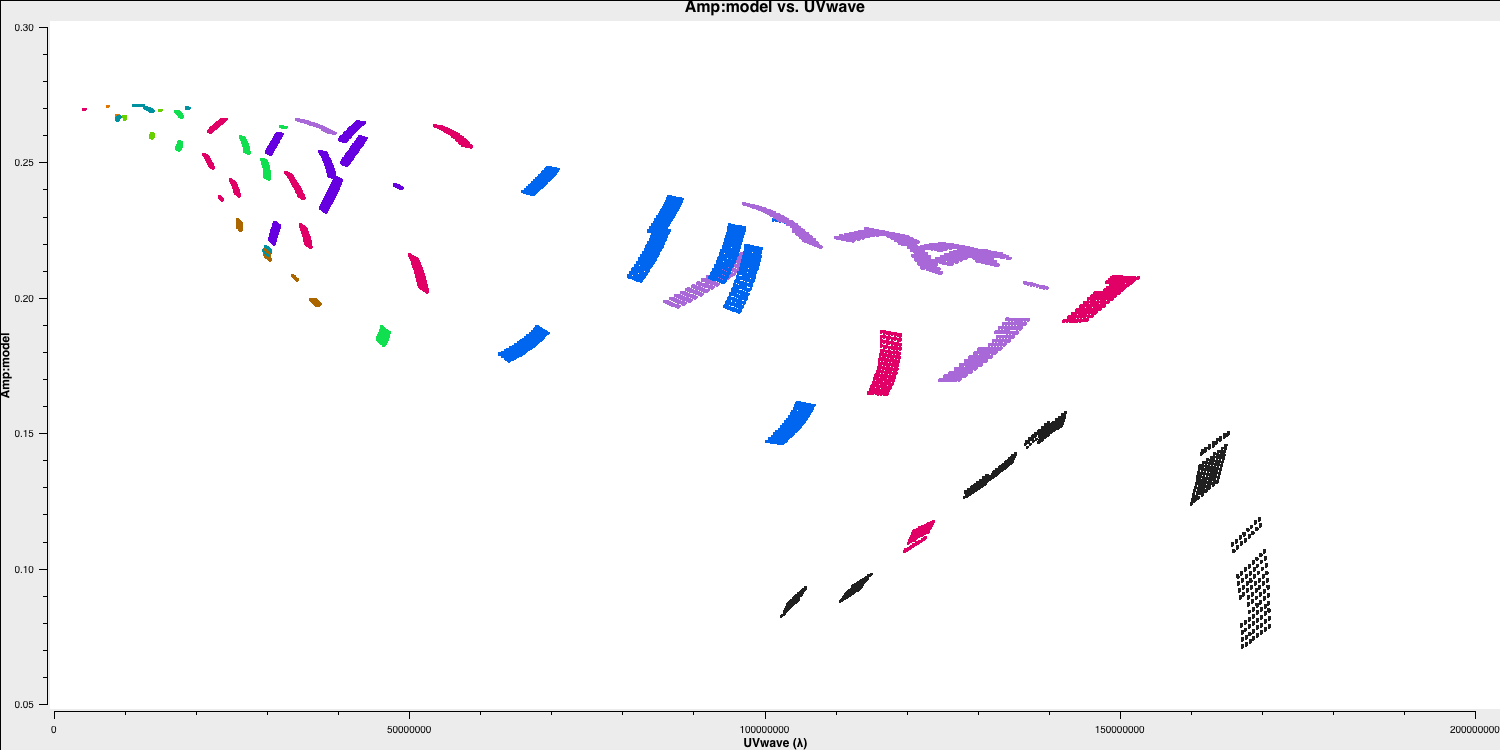In particular, you can see that on the west-east baselines, the source is mostly unresolved (i.e. flat across uv space). However, on the long baselines north-south to HH, the source is resolved. This is evident in the image with the faint jet to the north-west! Before imaging routines were developed, this is how radio astronomers used to derive source structure.

6F. Amplitude self-calibration

With the phases now in line, we want to correct any amplitude errors on our target source now that we have a large model S/N to play with. Remember from earlier, we want to increase the S/N per solution interval and a good rule of thumb is 3 x the phase-only solution interval.

• Look at step 18

### 18) Derive amplitude solutions
mystep = 18
if(mystep in thesteps):
print('Step ', mystep, step_title[mystep])

os.system('rm -rf '+target+'.ap')

gaincal(vis=target+'.ms',
caltable=target+'.ap',
corrdepflags=True,
solint='xx',
refant='xx',
gaintype='xx',
solnorm='xx',
calmode='xx',
gaintable=['xx'],
parang=False)

plotms(vis=target+'.ap',
gridrows=2,
gridcols=3,
yaxis='xxx',
xaxis='xxx',
iteraxis='xxx',
coloraxis='xxx')

applycal(vis=target+'.ms',
gaintable=['xx','xx'],
applymode='calonly',
parang=False)

• Input the appropriate parameters to obtain amp and phase solutions, plot them and apply them to the target source. Remember that we have already set the flux scale so we should normalize the amplitude solutions.
• Once you are happy then execute step 18
• Check the solutions to ensure they make sense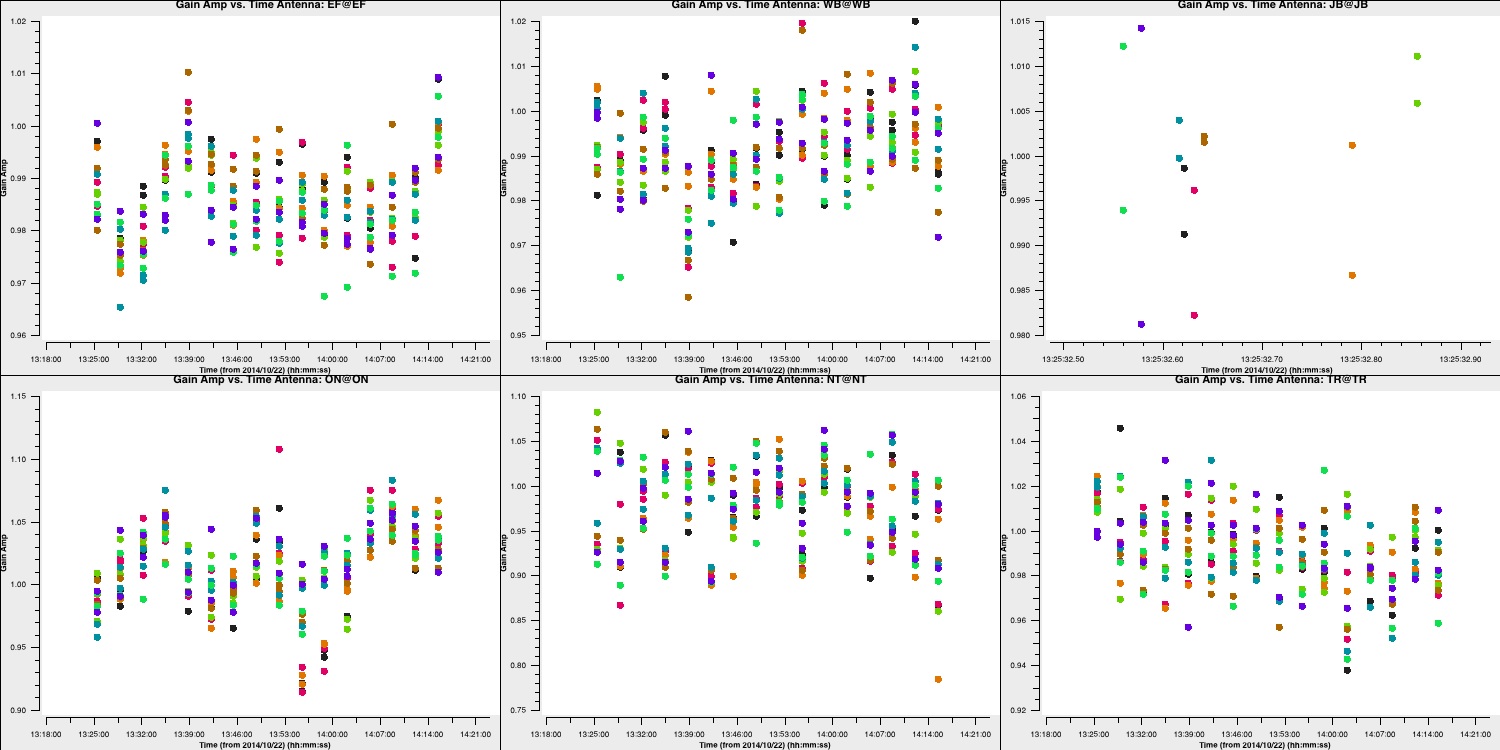6G. Final imaging

Now with the amplitude and phases corrected we want to image the source again to see if the amplitude solutions have improved our S/N. We would not expect a large change due to amplitude self-calibration as most amplitude errors are due to the antenna itself and this will be mostly accounted for by the phase calibrator amplitude calibration

• Look at step 19 and input the parameters into tclean and the imstat commands

### 19) Final imaging of target
mystep = 19
if(mystep in thesteps):
print('Step ', mystep, step_title[mystep])

os.system('rm -r '+target+'_sc_phase_amp*')
tclean(vis=target+'.ms',
imagename=target+'_sc_phase_amp',
stokes='pseudoI',
psfcutoff=0.5,
imsize=['xx','xx'],
cell=['xx'],
gridder='standard',
deconvolver='clark',
niter='xx',
interactive=True)

ft(vis=target+'.ms',
model=target+'_sc_phase_amp.model',
usescratch=True)

# Measure the signal to noise
rms=imstat(imagename=target+'_sc_phase_amp.image',
box='xx,xx,xx,xx')['rms']
peak=imstat(imagename=target+'_sc_phase_amp.image',
box='xx,xx,xx,xx')['max']

print('Peak %6.3f Jy/beam, rms %6.4f mJy/beam, S/N %6.0f' % (peak, rms*1e3, peak/rms))

• If you are happy, execute step 19. The last commands generates the amp/phase versis uv distance plots from before. The parameters for this have been set for you

I managed to get a large increase of S/N to $S_p = 0.244\,\mathrm{Jy\,beam^{-1}}$, $\sigma=0.202\,\mathrm{mJy\,beam^{-1}}$, $\mathrm{S/N}= 1205$.

• Using imview, open up the first target image and the self-calibrated images and see how the self-calibration process has improves the image fidelity and especially the noise profile

This concludes this section of the tutorial and you've made your first calibrated image of a target source, and learnt how to structure a simple calibration script. If you'd like to continue using this data then here are a few suggestions for extensions.

• Experiment with different weightings e.g. uniform, robust, to see if you can resolve the jet structure shown on the long N-S baselines
• Continue with self-calibration with finer solution intervals to see if you can push the S/N even further!

Otherwise, lets move onto part 3 where you shall make your own calibration script from scratch and calibrate our other phase-cal target pair we split out in part 1